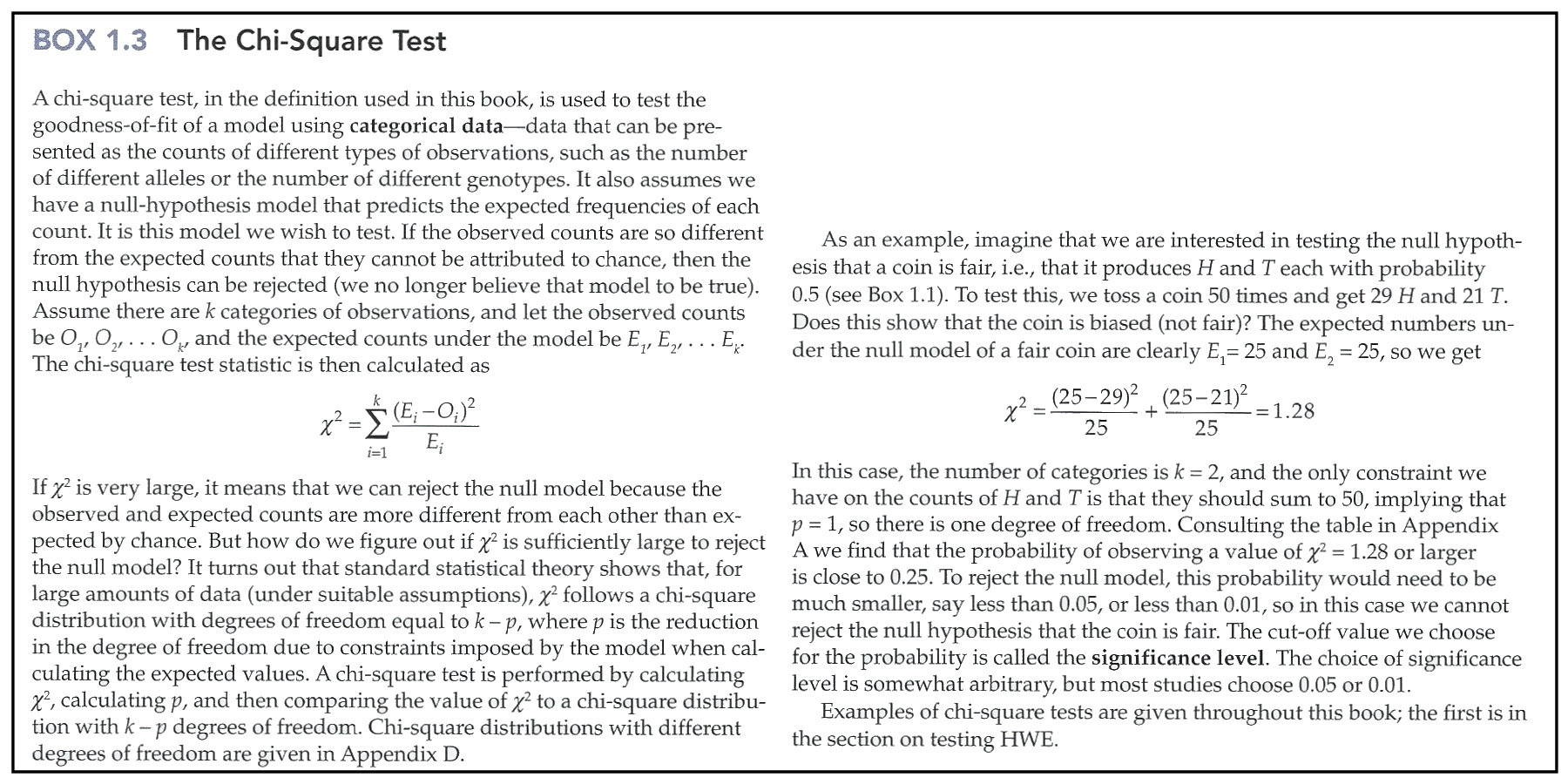The Chi-Square Test
[Table of Critical Values]

In combination with discussion of fundamentals, note in the above especially the concepts of Model, Categorical (Count) Data, Degrees of Freedom, & Significance Level. Consult the Table of Critical Values for a discussion of that concept.

HOMEWORK: The Power of a statistical test is related to the sample size necessary to detect what may be a small but significant deviation from expectation. The numbers presented show that with n = 50, an outcome of 29:21 is insufficient to demonstrate a significant deviation from equality. This begs the question, what outcome would be significant, or stated another way, what is the minimum deviation from expectation that could be detected with a sample of 50? From the formula above, and given a critical value of X2 = 3.84, show algebraically what that minimum deviation is for this simple case.

If you are feeling energetic, use Excel to calculate a table of the range of such values over a range of sample sizes 50, 100, 200, 500, and 1000. What does this tell you about the importance of sample size in testing biological hypotheses?

Customization of the Chi-Square Test for Nucleotide data

Box © 2013 Sinauer Associates; Text material © 2022 by Steven M. Carr